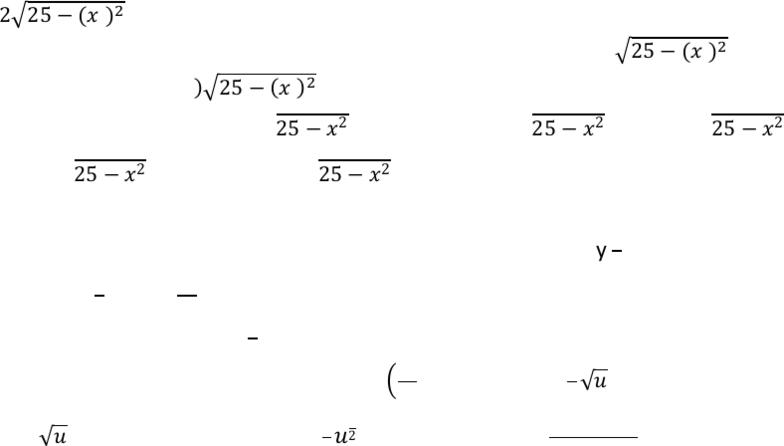Class Notes (1,100,000)
CA (620,000)
UTSG (50,000)
MAT (4,000)
MAT136H1 (900)
all (200)
Lecture

MAT136H1 Lecture Notes - Metric System, Pythagorean Theorem, Riemann Sum

Department
Mathematics
Course Code
MAT136H1
Professor
all

Page:
of 18.3 Challenging Integral Applications
Physics & Engineering Applications
Question #2 (Medium): Hydrostatic Force on a Submerged Vertical Plate
Strategy
The hydrostatic force is given by  
  
, where is the water density,
the depth below the surface,  the area of the thin th strip.
Sample Question
A vertical plate that is submerged in water has the shape shown by the diagram. Express the hydrostatic
force against one side of the place by a Riemann sum as well as an integral, and then evaluate it.
[a semi-circle with diameter of  meter submerged meter deep]
Solution
Since the units are given in the Standard International metric system,  
As for the th rectangular strip, the area is . If any th strip is chosen to be x distance from the
bottom of the semi-circle, then based on Pythagorean Theorem, the width (or even length) is:
. The distance the th strip is located beneath the water surface is
. Thus:  
    
  
 
 , and by the definition of definite integral, this is equal
to: 

 

 

 
. The first integral can be solved using
trigonometric substitution, however that would be the long tedious route. This represents the
top half of a circle with radius 5, but only over interval , so only
of the circle. The area
would then be

. For the second integral, it is in chain rule form, so let  ,
then   , and   
 Aligning the interval, when   , then  ; when
 , then     . Thus: 


  


 
  
   

Therefore, the hydrostatic force on the plate is .